Compressibility equation

The compressibility equation (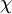$\chi$) can be derived from the density fluctuations of the grand canonical ensemble (Eq. 3.16 in Ref. 1). For a homogeneous system: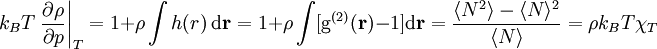$k_B T \left.\frac{\partial \rho }{\partial p}\right\vert_{T} = 1+ \rho \int h(r) ~{\rm d}{\mathbf r} = 1+\rho \int [{\rm g}^{(2)}({\mathbf r}) -1 ] {\rm d}{\mathbf r} = \frac{ \langle N^2 \rangle - \langle N\rangle^2}{\langle N\rangle}=\rho k_B T \chi_T$
where$p$ is the pressure,$T$ is the temperature,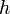$h$ is the total correlation function,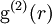${\rm g}^{(2)}(r)$ is the pair distribution function and$k_B$ is the Boltzmann constant.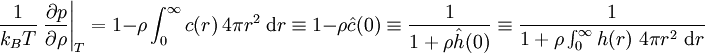$\frac{1}{k_BT} \left.\frac{\partial p}{\partial \rho}\right\vert_{T} = 1 - \rho \int_0^{\infty} c(r) ~4 \pi r^2 ~{\rm d}r \equiv 1- \rho \hat{c}(0) \equiv \frac{1}{1+\rho \hat{h}(0)} \equiv \frac{1}{ 1 + \rho \int_0^{\infty} h(r) ~4 \pi r^2 ~{\rm d}r}$